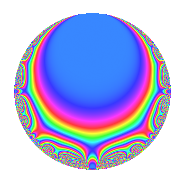# Properties

 Label 14.3.dLevel 14 Weight 3 Character orbit d Rep. character $$\chi_{14}(3,\cdot)$$ Character field $$\Q(\zeta_{6})$$ Dimension 4 Newforms 1 Sturm bound 6 Trace bound 0

# Related objects

## Defining parameters

 Level: $$N$$ = $$14 = 2 \cdot 7$$ Weight: $$k$$ = $$3$$ Character orbit: $$[\chi]$$ = 14.d (of order $$6$$ and degree $$2$$) Character conductor: $$\operatorname{cond}(\chi)$$ = $$7$$ Character field: $$\Q(\zeta_{6})$$ Newforms: $$1$$ Sturm bound: $$6$$ Trace bound: $$0$$

## Dimensions

The following table gives the dimensions of various subspaces of $$M_{3}(14, [\chi])$$.

Total New Old
Modular forms 12 4 8
Cusp forms 4 4 0
Eisenstein series 8 0 8

## Trace form

 $$4q$$ $$\mathstrut -\mathstrut 6q^{3}$$ $$\mathstrut -\mathstrut 4q^{4}$$ $$\mathstrut -\mathstrut 6q^{5}$$ $$\mathstrut +\mathstrut 8q^{7}$$ $$\mathstrut +\mathstrut O(q^{10})$$ $$4q$$ $$\mathstrut -\mathstrut 6q^{3}$$ $$\mathstrut -\mathstrut 4q^{4}$$ $$\mathstrut -\mathstrut 6q^{5}$$ $$\mathstrut +\mathstrut 8q^{7}$$ $$\mathstrut +\mathstrut 24q^{10}$$ $$\mathstrut +\mathstrut 18q^{11}$$ $$\mathstrut +\mathstrut 12q^{12}$$ $$\mathstrut -\mathstrut 36q^{14}$$ $$\mathstrut -\mathstrut 36q^{15}$$ $$\mathstrut -\mathstrut 8q^{16}$$ $$\mathstrut -\mathstrut 30q^{17}$$ $$\mathstrut -\mathstrut 24q^{18}$$ $$\mathstrut +\mathstrut 6q^{19}$$ $$\mathstrut +\mathstrut 54q^{21}$$ $$\mathstrut +\mathstrut 24q^{22}$$ $$\mathstrut +\mathstrut 30q^{23}$$ $$\mathstrut +\mathstrut 24q^{24}$$ $$\mathstrut +\mathstrut 4q^{25}$$ $$\mathstrut +\mathstrut 24q^{26}$$ $$\mathstrut -\mathstrut 20q^{28}$$ $$\mathstrut +\mathstrut 48q^{29}$$ $$\mathstrut -\mathstrut 12q^{30}$$ $$\mathstrut -\mathstrut 42q^{31}$$ $$\mathstrut -\mathstrut 90q^{33}$$ $$\mathstrut -\mathstrut 42q^{35}$$ $$\mathstrut -\mathstrut 62q^{37}$$ $$\mathstrut -\mathstrut 12q^{38}$$ $$\mathstrut +\mathstrut 12q^{39}$$ $$\mathstrut -\mathstrut 48q^{40}$$ $$\mathstrut +\mathstrut 72q^{42}$$ $$\mathstrut -\mathstrut 8q^{43}$$ $$\mathstrut +\mathstrut 36q^{44}$$ $$\mathstrut +\mathstrut 144q^{45}$$ $$\mathstrut +\mathstrut 36q^{46}$$ $$\mathstrut +\mathstrut 174q^{47}$$ $$\mathstrut -\mathstrut 20q^{49}$$ $$\mathstrut -\mathstrut 96q^{50}$$ $$\mathstrut +\mathstrut 54q^{51}$$ $$\mathstrut -\mathstrut 72q^{52}$$ $$\mathstrut -\mathstrut 78q^{53}$$ $$\mathstrut -\mathstrut 36q^{54}$$ $$\mathstrut +\mathstrut 48q^{56}$$ $$\mathstrut +\mathstrut 12q^{57}$$ $$\mathstrut +\mathstrut 24q^{58}$$ $$\mathstrut -\mathstrut 78q^{59}$$ $$\mathstrut +\mathstrut 36q^{60}$$ $$\mathstrut -\mathstrut 42q^{61}$$ $$\mathstrut -\mathstrut 216q^{63}$$ $$\mathstrut +\mathstrut 32q^{64}$$ $$\mathstrut -\mathstrut 84q^{65}$$ $$\mathstrut -\mathstrut 144q^{66}$$ $$\mathstrut -\mathstrut 58q^{67}$$ $$\mathstrut +\mathstrut 60q^{68}$$ $$\mathstrut +\mathstrut 84q^{70}$$ $$\mathstrut -\mathstrut 24q^{71}$$ $$\mathstrut -\mathstrut 48q^{72}$$ $$\mathstrut +\mathstrut 318q^{73}$$ $$\mathstrut +\mathstrut 96q^{74}$$ $$\mathstrut +\mathstrut 132q^{75}$$ $$\mathstrut +\mathstrut 126q^{77}$$ $$\mathstrut +\mathstrut 96q^{78}$$ $$\mathstrut +\mathstrut 110q^{79}$$ $$\mathstrut +\mathstrut 24q^{80}$$ $$\mathstrut +\mathstrut 18q^{81}$$ $$\mathstrut -\mathstrut 120q^{82}$$ $$\mathstrut +\mathstrut 12q^{84}$$ $$\mathstrut -\mathstrut 36q^{85}$$ $$\mathstrut +\mathstrut 24q^{86}$$ $$\mathstrut -\mathstrut 144q^{87}$$ $$\mathstrut -\mathstrut 24q^{88}$$ $$\mathstrut -\mathstrut 378q^{89}$$ $$\mathstrut +\mathstrut 24q^{91}$$ $$\mathstrut -\mathstrut 120q^{92}$$ $$\mathstrut -\mathstrut 138q^{93}$$ $$\mathstrut -\mathstrut 12q^{94}$$ $$\mathstrut -\mathstrut 30q^{95}$$ $$\mathstrut -\mathstrut 48q^{96}$$ $$\mathstrut -\mathstrut 120q^{98}$$ $$\mathstrut +\mathstrut 144q^{99}$$ $$\mathstrut +\mathstrut O(q^{100})$$

## Decomposition of $$S_{3}^{\mathrm{new}}(14, [\chi])$$ into irreducible Hecke orbits

Label Dim. $$A$$ Field CM Traces $q$-expansion
$$a_2$$ $$a_3$$ $$a_5$$ $$a_7$$
14.3.d.a $$4$$ $$0.381$$ $$\Q(\sqrt{2}, \sqrt{-3})$$ None $$0$$ $$-6$$ $$-6$$ $$8$$ $$q+\beta _{1}q^{2}+(-2-\beta _{1}-\beta _{2}+\beta _{3})q^{3}+\cdots$$